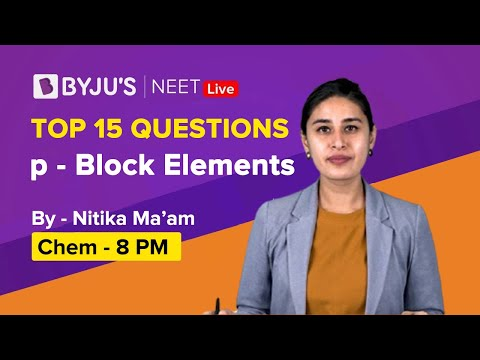Jet Set Go! All about Aeroplanes Jet Set Go! All about Aeroplanes

# Inorganic Chemistry Questions

Inorganic Chemistry is a major branch of chemistry that deals with compounds not containing carbon. Such compounds are called inorganic compounds. The synthesis, properties and uses of inorganic compounds are studied in inorganic chemistry. The most common example of an inorganic compound is sodium chloride (NaCl). The most essential molecule, water (H2O), that governs life is inorganic. Inorganic chemistry helps us to understand the chemistry of elements other than carbon.

 Definition: The first organic compound synthesized in the laboratory was Urea (NH2CONH2) from an inorganic compound Ammonium Chloride (NH4Cl). Alfred Werner is known as the father of inorganic chemistry. His work on coordination chemistry was awarded the Nobel Prize in 1913.

## Inorganic Chemistry Questions with Solutions

Q1. What is the formula of blue vitriol?

1. CuSO4.4H2O
2. CuSO4.5H2O
3. CuSO4.6H2O
4. CuSO4.7H2O

Copper (II) Sulfate is also known as blue vitriol. It is used as an insecticide in agriculture.

Q2. Name few essential inorganic elements for our human body.

Answer: The essential inorganic elements for our human body are Magnesium (Mg), Sodium (Na), Potassium (K), Calcium (Ca).

Q3. Explain Isomerism.

Answer: Compounds which have the same molecular formula but differ in their arrangement of atoms in space are called isomers and the property is called isomerism. For example, cisplatin. It has two isomeric forms, the cis form and the trans form. The figure below shows cis and trans platin (left- cis platin, right- trans platin). The cis form is used in cancer therapy.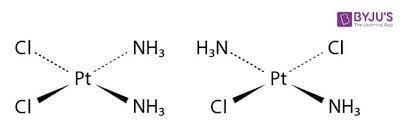Q4. According to Werner’s theory, how many types of valencies do metal complexes have?

Answer: Metal complexes have two types of valencies, a primary valency and a secondary valency. Primary valency corresponds to the oxidation state of the metal ion and secondary valency corresponds to the coordination number of the metal ion.

Q5. List the different types of isomerism in coordination complexes.

Answer: The different types of isomerism in coordination complexes are:

1. Ionization Isomerism
2. Coordination Isomerism
4. Geometrical isomerism
5. Optical isomerism

Q6. Give two examples of ambidentate ligands.

Answer: Thiocyanate (-SCN) is an ambidentate ligand that can bind to the metal center (M) either by sulfur atom or by nitrogen atom. Nitrito (NO2) is another example of ambidentate ligand as it can bind to the metal center (M) either by nitrogen atom or by oxygen atom.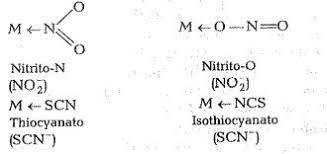Q7. Match the following items of column 1 with column 2 and choose the correct answer:

Column 1 (Elements)

Column 2 (Uses)

1) Chlorine

a) Batteries

2) Neon

b) Required for strong bones

3) Calcium

c) Water disinfection

4) Lithium

d) Bulbs

Column 1

Column 2

1) Chlorine

c) Water disinfection

2) Neon

d) Bulbs

3) Calcium

b) Required for strong bones

4) Lithium

a) Batteries

Q8. Match the following items of column 1 with column 2 and choose the correct answer:

Column 1

Column 2

1) HCl

a) Base

2) NaCl

b) Acid

3) Ca(OH)2

c) Salt

Column 1

Column 2

1) HCl

b) Acid

2) NaCl

c) Salt

3) Ca(OH)2

a) Base

Q9. Calculate the pH of HCl acid when the concentration of [H]+ ions is 0.1 M.

Answer: pH is calculated by the formula:

pH = – log [H]+

pH = – log (0.1)

pH = 1

Therefore the pH of HCl acid is 1.

Q10. Alkali and Alkaline earth metals belong to which group of periodic table?

Answer: Alkali and Alkaline Earth metals belong to group 1 and group 2 of the periodic table respectively. They are collectively called s-block elements.

Q11. Which compound is called as inorganic benzene?

1. B3H6N3
2. B3H3N3
3. BH3N3
4. B6H3N3

B3H6N3 is called as inorganic benzene or Borazine.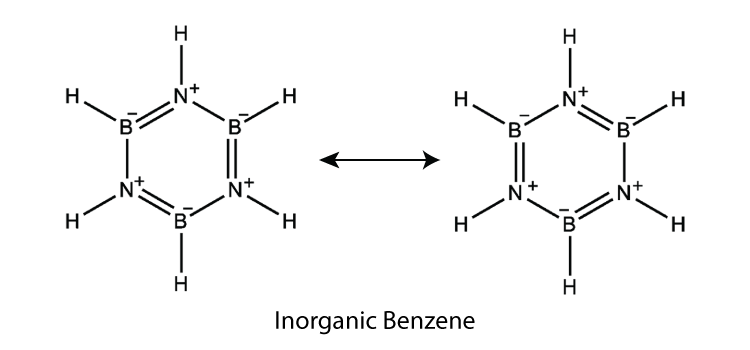Q12. Calculate the pOH of base NaOH having a concentration of 1 ✕ 10-10M.

pOH = – log [OH]

pOH = – log (1 ✕ 10-10 )

pOH = -(-10 log 10)

pOH = 10 log 10

pOH = 10 ✕ 1

pOH = 10

The pOH of NaOH is 10.

Q13. What are Arrhenius acids and Bases? Give examples.

Answer: The acids which give H+ ions in water are called Arrhenius acids. The bases which give OH ions in water are called Arrhenius bases. For example, CO2 is an Arrhenius acid and CaO is an Arrhenius base.

CO2 + H2O → H2CO3 → 2H+ + CO32-

CaO + H2O → Ca(OH)2 → Ca2+ + 2OH

Q14. What are Bronsted Lowry acids and bases?

Answer: Proton (H+) donors are called Bronsted Lowry acids and proton acceptors are called Bronsted Lowry bases.

Q15. Give an example of Lewis acid.

Answer: BF3 is an example of lewis acid. It is an electron pair acceptor.

## Practice Questions on Inorganic Chemistry

Q1.What is the chemical formula of diborane? What is its structure?

Q2. How many banana bonds are there in diborane?

Q3.What is the HSAB principle?

Q4. What is the geometry and shape of the SF4 molecule?

Q5.What is the hybridization of methane?

Click the PDF to check the answers for Practice Questions.

## Recommended Videos

#### Chemical Bonding Introduction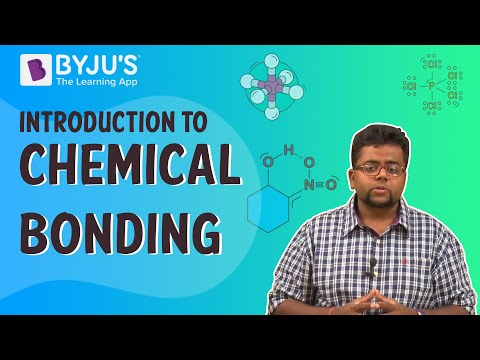#### P Block Elements Questions